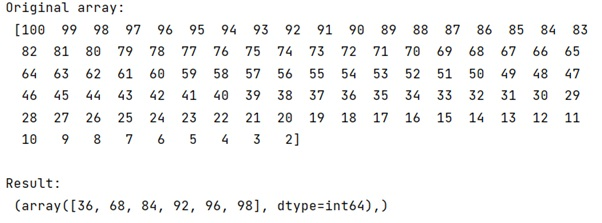# How to turn a boolean array into index array in numpy?

Learn, how to turn a boolean array into index array in numpy in Python?
Submitted by Pranit Sharma, on January 15, 2023

NumPy is an abbreviated form of Numerical Python. It is used for different types of scientific operations in python. Numpy is a vast library in python which is used for almost every kind of scientific or mathematical operation. It is itself an array which is a collection of various methods and functions for processing the arrays.

## Turning a boolean array into index array in numpy

Suppose that we are given a NumPy array and we need to focus on finding an efficient way to retrieve the integer indexes of locations in an array based on a condition that is true as opposed to the Boolean mask array.

We will use np.where() for this purpose and pass our specific condition to create a mask of values.

Let us understand with the help of an example,

## Python code to turn a boolean array into index array in numpy

```# Import numpy
import numpy as np

# Creating a numpy array
arr = np.arange(100,1,-1)

# Display original array
print("Original array:\n",arr,"\n")

res = np.where(arr&(arr-1) == 0)

# Display result
print("Result:\n",res)
```

Output: